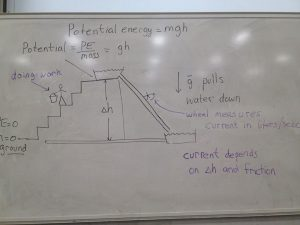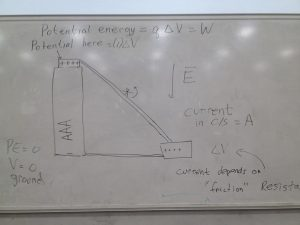# What do you need to know about Electric Charge and Field?

## 30 Jul What do you need to know about Electric Charge and Field?

a

There are only four fundamental forces in physics, and in high school physics we will learn about three of them.  These forces are called “fundamental” because we don’t have a deeper explanation for why they exist.  The four are

1. Gravitational Force
2. Electric Force
3. Strong nuclear force

a

## Gravitational force

Gravity is the most familiar of the four.  Even though gravity is relatively weak, it has a big impact on us because it is an attraction between masses, and we happen to spend our entire lives in very close proximity to an enormous, enormous mass:  planet Earth.

aa

Gravity is so familiar to us that humans observed its effects – things fall down –  for thousands of years before anyone really recognized it for what it is.

a

Isaac Newton was the one to realize that the force that makes apples fall from trees is the same force that makes the moon travel in an elliptical (nearly circular) path around the earth, and the earth follow an elliptical (nearly circular) path around the sun.

a

Newton deduced that gravitational attraction between point masses must follow an inverse square law because that’s how you get elliptical orbits, and he knew that Kepler had already determined that the orbits of the planets were all elliptical.

In studying gravitational fields, we learn that gravitational fields are responsible for the force that we call weight, and we can calculate weight using F = mg.

We learned Newton’s law,

a

### F = GMm/r^2

a

It tells us the force of attraction between two point masses, or between two spherical masses, if we measure from the center of one sphere to the center of the other.

aa

We learned that we can use the concept of an invisible field to understand action at a distance, and we learned to characterize the strength of that field by considering the force per unit of mass that it exerts on a test mass placed in the field.

We learned that because massive objects have weight, they gain potential energy when we displace them in opposition to a gravitational field.  That’s a fancy way to say that the object gains energy when we lift it up.  delta PE = m*g*delta h, where h is the height of the object.

a

What does all this have to do with electric charge and electric fields?

a

## Electric field

The easiest way to build your understanding of electric fields is to recognize how similar they are to gravitational fields. Once you know the concepts of one, you know the concepts of the other.  When you can analyze one, you can analyze both.

a

Gravity is an attraction between masses.  What is mass?  It is a fundamental property of a particle or object.  It tells us how hard gravity will pull on that object.

The electric force is just like gravity, but instead of operating on mass, it operates on another fundamental property that a particle or object has: charge.  Charge tells us how hard the electric field will pull on an object.a

Like gravity, the electric force follows an inverse square law that has exactly the same form as Newton’s law.  We just replace mass with charge.

a

We define electric field strength as the force per unit of charge it exerts on a positive test charge placed in the field.a

a

That points to an important difference between gravitational force and electric force:

• There’s only one kind of mass, but there are two kinds of charge.

a

• We’ve arbitrarily named them positive and negative, but we could have just as well called them red and blue.

a

• Unlike charges attract, the way masses attract each other with gravity, but like charges repel each other, and we don’t see that kind of effect with gravity.

a

Here now is a copy of the paragraph above about the gravitational force, adjusted so that it is about the electric force:

a

In studying ELECTRIC FIELDS, we learn that ELECTRIC FIELDS are responsible for the force BETWEEN TWO CHARGES, and we can calculate THAT FORCE using:

### F = qE

aWe learned COULOMB’s law,  F = kQq/r^2.  It tells us the force of attraction between two point CHARGES, or between two spherical CHARGES, if we measure from the center of one sphere to the center of the other.

a

We learned that we can use the concept of an invisible field to understand action at a distance, and we learned to characterize the strength of that field by considering the force per unit of CHARGE that it exerts on a test CHARGE placed in the field.

a

We learned that because CHARGED objects EXPERIENCE ELECTRIC FORCE, they gain potential energy when we displace them in opposition to an ELECTRIC field. That’s a fancy way to say that the object gains energy when we MOVE IT THROUGH A POTENTIAL DIFFERENCE.  delta PE = m*delta V, where V is the ELECTRIC POTENTIAL AT THE LOCATION of the object.

a

See the similarity? Gravity is more intuitive because you experience it all the time, but the electric force works mostly the same way on charges as gravity works on masses.

a

There are some differences too. There are two types of charges but only one type of mass, so the electric force can repel or attract, while gravity can only attract.

a

The electric force is much, much stronger than the gravitational force.  How can we compare them?  Consider the magnitude of the electric force between two electrons, and compare it to the gravitational force between them.

The electric force is more than 40 orders of magnitude larger!  Ironically, the strength of the electric force is the reason we rarely notice it.  Because it is so strong, positive and negative charges are almost always paired together, so most objects are neutral, making it hard for us to notice the effect.

But consider what happens when you pull a comb through your hair, or rub a balloon with some fur.  You can use the comb or balloon to pick up scraps of paper. That might not seem so impressive until you realize that the little charge on the comb or balloon is exerting a force on the paper that is stronger than the gravitational force exerted by on the paper by the entire planet!

aa

The electric force is so strong that we don’t notice that is is actually the force that is at work when we push or pull on something.  What we think of as touch is really a manifestation of the electric force!  When we push on a chair, if we look at a microscopic level, what’s really going on is that the electrons in our hand are repelling the electrons in the book.  Our atoms are neutral but the electrons are on the outside and the protons are on the inside, so if you bring atoms close together the way they bump into each other is via the repulsion of their electrons.

a

I like to make a chart to show the similarities (and differences) between gravity and the electric force

This illustration helps complete the analogy between the two types of fields:I love this clip of Richard Feynman talking about the electric force

Here’s a nice video that helps visualize the shape of the electric field

,